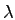## 5. 2. 1 Class Layout

Usually the coefficients are floating point numbers. In order to offer the choice of the data type to the user, templating is used. For this reason, the class can be schematically written in the following way:

 template<typename NumericT>
class linearized_expression
{
typedef linearized_expression<NumericT> self;
typedef std::map<int, NumericT>::iterator map_iter_t;

std::map<int, NumericT> coefficients;

std::map<int, NumericT> lambda_coefficients;
... or ...
NumericT right_hand_side;
};


This snippet shows the implementation for both, the eigenvalue expression as well as the linear (affine) expression. In one case allmultiplied variables are stored in a separate map.

Michael 2008-01-16